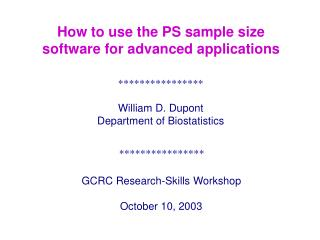DownloadDownload Presentation****************

# ****************

Télécharger la présentation## ****************

- - - - - - - - - - - - - - - - - - - - - - - - - - - E N D - - - - - - - - - - - - - - - - - - - - - - - - - - -
##### Presentation Transcript

1. GCRC Research-Skills Workshop October 10, 2003 How to use the PS sample size software for advanced applications **************** William D. Dupont Department of Biostatistics ****************

2. Follow-up time Fate at exit Statistic: Log-rank test Power: Schoenfeld & Richter, Biometrics 1982 Survival Analysis Accrual time A Additional follow-up F Sample Size 0 Time

3. Relative Risk (Hazard Ratio) for controls relative to experimental subjects Median survival for experimental subjects Median survival for controls = Median Survival 6 3 = = 2 Experimental treatment Control treatment

4. For t tests power calculations for increased or decreased response relative to control response are symmetric. i.e. The power to detect is the same as the power to detect This is not true for Survival Analysis. The power to detect a two-fold increase in hazard does not equal the power to detect a 50% decrease in hazard.

5. Power to detect treatment 1 vs. control greater than treatment 1 vs.control Treatment 1 Control Relative Risk Treatment 1 vs Control 0.5 Treatment 2 vs. Control 2 Control vs. Treatment 1 2 Treatment 2

6. Multiple power curves can be plotted on a single graph. Black and white plots can be produced. It is important to read the parameter definitions carefully.

7. Power Calculations for Linear Regression

8. s = 4.75 x 45 · · 40 · · · · · · · 35 · · · · · · · · Birthweight (g/100) · · · · · · 30 · · · · · · · 25 · 20 5 10 15 20 25 30 Estriol (mg/24 hr) Rosner Table 11.1 Am J Obs Gyn 1963;85:1-9 = standard deviation of x variable = standard deviation of y variable

9. = standard deviation of the regression errors Estimated by s= root mean squared error (MSE)

10. r = -1 r = 1 r = 0 0 < r < 1 c) Correlation coefficient is estimated by {1.2}

11. 35 Treatment r A 0.9 B 0.6 30 25 20 Response Variable y Both treatments have identical values of mx = 6, my = 20 sx = 2, sy = 5 15 10 5 1 3 5 6 7 8 9 10 11 12 2 4 Independent Variable x Measures of association between continuous variables Correlation vs. linear regression

12. Treatment l r s A 2.25 0.9 2.18 B 1.5 0.6 4.0 35 30 25 20 Response Variable y Both treatments have identical values of mx = 6, my = 20 sx = 2, sy = 5 15 10 5 1 3 5 6 7 8 9 10 11 12 2 4 Independent Variable x

13. th j Regression Error • y j l g + l x j 1 Unit Patient Response of Dosage g Regression g + l x Line x 0 j Treatment Dose Level

14. c) Slope parameter estimate l (a.k.a. ) is estimated by b = r sy /sx

15. r = -1 r = 1 r = 0 0 < r < 1 l is estimated by b = r sy /sx

16. Studies can be either experimental or observational

17. Normally distributed unless investigator chooses level Chosen by investigator Treatment level

18. Estimating s directly is often difficult If we can estimate or s = = s Warning: If the anticipated value of in your experiment is different from that found in the literature then your value of r will also be different.

19. 35 30 25 Response Variable y 20 15 10 5 1 3 5 6 7 8 9 10 11 12 2 4 Independent Variable x

20. Relationship between BMI and exercise time n = 100 women willing to follow a diet exercise program for six months Interquartile range (IQR) of exercise time = 15 minutes (pilot data) = (IQR / 2) / z0.25 = 7.5 / 0.0675 = 11.11 = 4.0 kg/m2 = standard deviation of BMI for women obtained by Kuskowska-Wolk et al. Int J Obes 1992;16:1-9 Would like to detect a true drop in BMI of = -0.0667 kg/m2 per minute of exercise (1/2 hour of exercise per day induces a drop of 2 kg/m2 over 6 months)

21. Interquartile Range In general = (IQR / 2) / z0.25 When s = 1 0.25 0.25 2 0 -2 -0.675 0.675

22. Impaired Antibody Response to Pneumococcal Vaccine after treatment for Hodgkin’s Disease Siber et al. N Engl J Med 1978;299:442-448. n = 17 patients treated with subtotal radiation. vaccinated 8 to 51 months later A linear regression of log antibody response against time from radiation to vaccination gave Suppose we wanted to determine the sample size for a new study with patients randomized to vaccination at 10, 30, or 50 weeks

23. Composing Slopes of Two Linear Regressin Lines Armitage and Berry (1994) gave age and pulmonary vital capacity for 28 cadmium industry workers with > 10 years of exposure 44 workers with no exposure

24. (unexposed) (exposed) pooled error variance How many workers do we need to detect with ratio of unexposed workers m = 44/28 = 1.57? Need 167 exposed workers and 167 x 1.57 = 262 unexposed workers